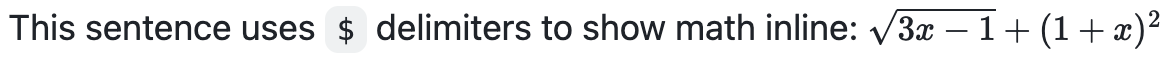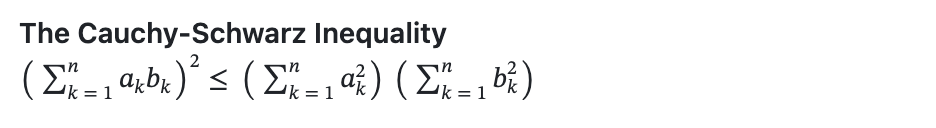# Writing mathematical expressions

## ここには以下の内容があります:

Use Markdown to display mathematical expressions on GitHub.

To enable clear communication of mathematical expressions, GitHub supports LaTeX formatted math within Markdown. For more information, see LaTeX/Mathematics in Wikibooks.

GitHub's math rendering capability uses MathJax; an open source, JavaScript-based display engine. MathJax supports a wide range of LaTeX macros, and several useful accessibility extensions. For more information, see the MathJax documentation and the MathJax Accessibility Extensions Documentation.

## Writing inline expressions

To include a math expression inline with your text, delimit the expression with a dollar symbol $. This sentence uses $ delimiters to show math inline:  $\sqrt{3x-1}+(1+x)^2$## Writing expressions as blocks

To add a math expression as a block, start a new line and delimit the expression with two dollar symbols $$. **The Cauchy-Schwarz Inequality**$$\left( \sum_{k=1}^n a_k b_k \right)^2 \leq \left( \sum_{k=1}^n a_k^2 \right) \left( \sum_{k=1}^n b_k^2 \right)## Writing dollar signs in line with and within mathematical expressions

To display a dollar sign as a character in the same line as a mathematical expression, you need to escape the non-delimiter $ to ensure the line renders correctly. • Within a math expression, add a \ symbol before the explicit $.

This expression uses \$ to display a dollar sign:$\sqrt{\$4}$• Outside a math expression, but on the same line, use span tags around the explicit $. To split <span>$</span>100 in half, we calculate $100/2$